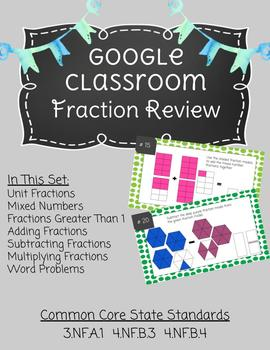# Fraction Review - DigitalSubject
Resource Type
File Type
PDF (475 KB|40 pages)
Standards
\$5.00
• Product Description
• Standards
Looking for a quick fraction review that needs little prep? This fraction review can be pushed out to students via google classroom requiring no copies!

Included in the review:
- Unit fractions
- Mixed number fractions
- Fractions greater than 1
- Subtracting fractions
- Multiplying fractions
- Word problems with fractions

Before each set of review questions there is an overview slide that tells what the section is about and gives the students an example!

Please rate this product and leave feedback to earn TPT credits for your next purchase!
Apply and extend previous understandings of multiplication to multiply a fraction by a whole number.
Solve word problems involving addition and subtraction of fractions referring to the same whole and having like denominators, e.g., by using visual fraction models and equations to represent the problem.
Add and subtract mixed numbers with like denominators, e.g., by replacing each mixed number with an equivalent fraction, and/or by using properties of operations and the relationship between addition and subtraction.
Decompose a fraction into a sum of fractions with the same denominator in more than one way, recording each decomposition by an equation. Justify decompositions, e.g., by using a visual fraction model. Examples: 3/8 = 1/8 + 1/8 + 1/8; 3/8 = 1/8 + 2/8; 2 1/8 = 1 + 1 + 1/8 = 8/8 + 8/8 + 1/8.
Understand addition and subtraction of fractions as joining and separating parts referring to the same whole.
Total Pages
40 pages